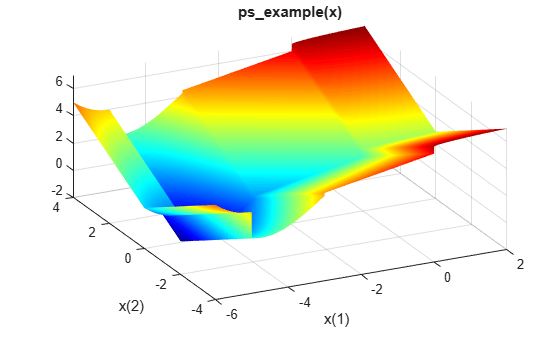# Optimize Nonsmooth Function Using `patternsearch`, Problem-Based

This example shows how to minimize a nonsmooth function using direct search in the problem-based approach. The function to minimize, `ps_example(x)`, is included with Global Optimization Toolbox software.

Plot the objective function.

```fsurf(@(x,y)reshape(ps_example([x(:),y(:)]),size(x)),... [-6 2 -4 4],"LineStyle","none","MeshDensity",300) colormap 'jet' view(-26,43) xlabel("x(1)") ylabel("x(2)") title("ps\_example(x)")```Create a 2-D optimization variable `x`. The `ps_example` function expects the variable to be a row vector, so specify `x` as a 2-element row vector.

`x = optimvar("x",1,2);`

To use `ps_example` as the objective function, convert the function to an optimization expression using `fcn2optimexpr`.

`fun = fcn2optimexpr(@ps_example,x);`

Create an optimization problem with objective function `ps_example`.

`prob = optimproblem("Objective",fun);`

Specify the initial point `x0` as a structure with field `x` taking the value `[2.1 1.7]`.

`x0.x = [2.1 1.7];`

Solve the problem, specifying the `patternsearch` solver.

`[sol,fval] = solve(prob,x0,"Solver","patternsearch")`
```Solving problem using patternsearch. Optimization terminated: mesh size less than options.MeshTolerance. ```
```sol = struct with fields: x: [-4.7124 -7.6294e-07] ```
```fval = -2.0000 ```

`patternsearch` finds a better solution (lower function value) than the default `fminunc` solver, which is not recommended for minimizing nonsmooth functions.

`[solfminunc,fvalfminunc] = solve(prob,x0)`
```Solving problem using fminunc. Local minimum possible. fminunc stopped because it cannot decrease the objective function along the current search direction. ```
```solfminunc = struct with fields: x: [1.9240 8.8818e-16] ```
```fvalfminunc = 2.9161 ```